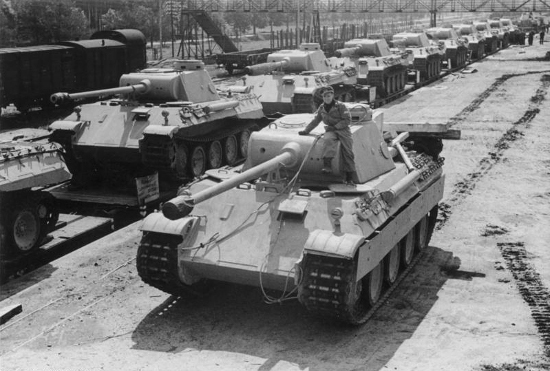# The German Tank ProblemDuring World War II, as they mulled whether to attempt an invasion of the continent, the Allies needed to estimate the number of tanks Germany was producing. They asked their intelligence services to guess the number by spying on German factories and counting tanks on the battlefield, but these efforts produced contradictory estimates. Finally they resorted to statistical analysis.

They did this by studying the serial numbers on captured and destroyed German tanks. Suppose German tanks are numbered sequentially 1, 2, 3, …, B, where B is the total number of tanks that we seek to know. And suppose that we have five captured tanks whose serial numbers are 21, 35, 42, 60, and 89. It turns out that$\displaystyle B = \frac{(N+1)M}{N} - 1,$

where N is the sample size (here, 5) and M is the highest sampled number (here, 89). In this example, the formula tells us that B = 105.8, so we’d estimate that 106 tanks had been produced at that time.

In the event, Allied statisticians reportedly estimated that the Germans had produced 246 tanks per month between June 1940 and September 1942. Intelligence estimates had put the total at about 1,400. When the Allies captured German production records after the war, they found that they had produced 245 tanks per month during those three years, almost precisely what the statisticians had predicted, and less than 20 percent of the intelligence estimate.

(Thanks, Ryan.)